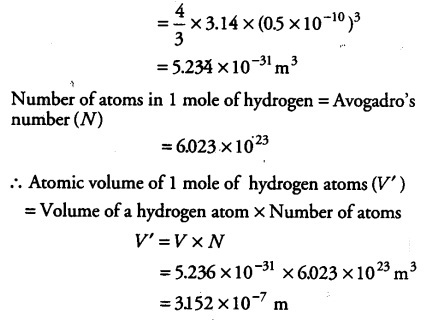# What is the total atomic volume in ${{m}^{3}}$ of a mole of hydrogen atoms?

The unit of length convenient on the atomic scale is known as an angstrom and is denoted by A°. 1 A° = ${{10}^{-10}}$ m. The size of the hydrogen atom is about 0.5 A°. What is the total atomic volume in ${{m}^{3}}$ of a mole of hydrogen atoms?

Radius of a hydrogen atom ® = 0.5 A° = 0.5 x {{10}^{-10}} m
Volume of each hydrogen atom (V) = 4/3 \pi {{r}^{3}}